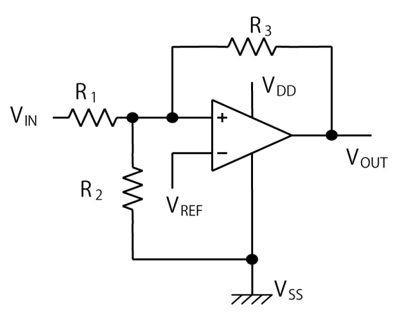# [Comparator] How do I set hysteresis in a comparator?

Here are some examples of how to set the hysteresis voltage of a comparator.
Conditions: VDD = 3.3 V, VREF = 1.4 V, hysteresis width = 6 mV, rise detection voltage 1.650 V

1) R3 rate
In order to minimize the influence of the input leakage voltage, it is necessary to pass a current of at least 0.5 µA to R3. The current flowing to R3 is (VREF − VOUT)/IR3, with VOUT = 0 V or VOUT = VDD, so you can obtain the rate by calculating R3 = VREF/IR3 or R3 = (VDD − VREF)/IR3.
The smaller of the two results is used. When VDD = 3.3 V, VREF = 1.4 V and IR3 = 1 µA, the two resistance rates are 1.4 MΩ and 1.9 MΩ, so our choice here is 1.4 MΩ.

2) Hysteresis width
VHB will be 6 mV in this case.

3) R1 rate
R1 = R3 (VHB/VDD)
R1 = 1.4M (6m/3.3) = 2.55 kΩ

4) VTHR
Set the rise detection point of VTHR so that VTHR > VREF(R1 + R3)/R3 is satisfied. It will be 1.650 V in this case.

5) R2 rate
R2 = 1/(VTHR/(VREF⋅R1) − (1/R1) − (1/R3))
= 1/(1.65/(1.4⋅2.55k) − (1/2.55k) − (1/1.4M))
= 14.4 kΩ

6) Result
The rise and fall time and hysteresis width are as follows:
Rise time: VTHR = VREF⋅R1(1/R1 + 1/R2 + 1/R3)
Fall time: VTHF = VTHR − R1⋅VCC/R3
Hysteresis: VHB = VTHR − VTHF = R1⋅VCC/R3# [Comparator] The rise time of the output waveform in the S-89220 (comparator) is much longer than that described in the data sheet (35 ms vs. 2 µs). Why is this?

This might happen when you create your own circuit and set the common-mode input voltage of the comparator to a value that exceeds the level specified in our data sheet (i.e., a value that approaches VDD). Please be aware that the common-mode input voltage of the S-891 series and S-892 series is not full range from VSS to VDD.

# [Op Amp / Comparator] How do we handle the unused operational amplifier (or comparator) of dual products?

The device will not be damaged if you leave input pins open but it makes the performance unstable.
The characteristics of the operational amplifier are also affected on the user side. Thus we recommend you to implement the following procedure.
Operational amplifier:
Set a voltage follower configuration and connect the input Vin+ to VSS.
Comparator:
Connect Vin+ to VSS and Vin− to VDD.
If, however, you want to use only one circuit of a dual product, we recommend using a single product.

# [Op Amp / Comparator] How much is the maximum value of the input offset current?

The input offset current is in the order of 1pA because it flows through the gate oxide of the MOS transistor. However, it is difficult to measure it correctly because of the leakage current of the package pins or because the measurement accuracy is in the pA order. Under our test conditions, the S-891 series and S-892 series are tested whether the maximum value of the input offset current is 50 nA or less. Other mini analog products are tested whether this maximum value is 10 nA or less.

# [Op Amp / Comparator] Is the device damaged if the input voltage exceeds VDD, or falls below VSS?

The data sheet describes that the maximum rating of the input voltage for mini analogs is VSS to VDD.
This is the range of input voltage that is guaranteed by our reliability test data.

However, the device should not be damaged even if you apply an input voltage a little outside this range. How much outside this range is allowable can be defined as follows:
As described in the data sheet there is an off-transistor (NMOS) connected between the input pins and VSS for protection against ESD. The current increases when the input voltage falls below VSS – 0.7 V when you apply a voltage of less than VSS to the input pins. With a voltage down to VSS – 0.5 V, damage should not occur.

A current pass does not exist between the input pins and VDD because there is no protection device between them. The device should not be damaged as long as the input voltage is less than VSS + 7 V when you apply a voltage of more than VDD to the input pins.

# [Op Amp / Comparator] What does an equivalent circuit of each pin look like?

It depends on the individual product.
Please refer to our data sheet.

# [Op Amp / Comparator] What does a protection circuit look like?

It depends on the invidual product. Please refer to our data sheet.

• Tips and hints described herein are for your reference only. ABLIC shall not be liable for any warranty and responsibility of use or application of ABLIC's ICs in or to your product regardless of description herein.
• No part of this document may be reproduced or transcribed in any form for any purpose without the permission of ABLIC.
• Information described herein is subject to revision or change without notice.